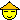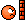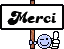# Importer un indicateur sur ProRealTime

Pour discuter sur l’interface de ProRealTime Software, nos configurations graphiques...
Bonjour,

J'ai téléchargé le code d'un indicateur pour ProRealTime, mais je n'arrive pas à l'installer.
Un simple indicateur basé sur les volumes.
J'ai simplement copier/coller le code dans ProBuilder mais cela ne fonctionne pas. Il semble qu'une erreur de syntaxe intervienne.
N'y connaissant rien en programmation, je me permets de poster ici pour demander de l'aide.

Merci.
Cordialement.

Le fichier au format pdf :

Voici le code débarrassé des erreurs de syntaxesN'oublie pas de créer les 3 variables :

History = Boolean
Use2Bars = Boolean
Lookback = 20

Code: Tout sélectionner
`// Better Volume Indicator - Summary// ClimaxUp (Red) : Top, Start Up Trend, Down Trend Continue// ClimaxDown (White): Bottom, Up Trend Continue, Start Down Trend// LowVolume (Yellow): Bottom, Top, Up Trend Continue// Churn (Green): Bottom, Top, Down Trend Continue// ClimaxChurn (Magenta): Top, Down Trend Continue// Parameter: History = Boolean// Parameter: Use2Bars = Boolean// Parameter: Lookback = 20ONCE Condition1 = 0ONCE Condition2 = 0ONCE Condition3 = 0ONCE Condition4 = 0ONCE Condition5 = 0ONCE Condition6 = 0ONCE Condition7 = 0ONCE Condition8 = 0ONCE Condition9 = 0ONCE Condition10 = 0ONCE Condition11 = 0ONCE Condition12 = 0ONCE Condition13 = 0ONCE Condition14 = 0ONCE Condition15 = 0ONCE Condition16 = 0ONCE Condition17 = 0ONCE Condition18 = 0ONCE Condition19 = 0ONCE Condition20 = 0VolValue = VolumeLowVolValue = VolValueClimaxUpValue = VolValueClimaxDownValue = VolValueChurnValue = VolValueClimaxChurnValue = VolValueStopVolValue = VolValueTrampolineValue = VolValueAVVolume = Average[Lookback*2](VolValue)IF History = 0 THENBI = 400ELSIF History = 1 THENBI = LookbackENDIFIF BARINDEX > BI AND Volume <> 0 THENIF Close > Open THENValue1 = Volume * (Range / (2 * Range + Open - Close))ELSIF Close < Open THENValue1= Volume * ((Range + Close - Open) / (2 * Range + Close - Open))ENDIFIF Close = Open THENValue1 = 0.5 * VolumeENDIFValue2 = Volume - Value1Value3 = Value1 + Value2Value4 = Value1 * RangeValue5 = (Value1 - Value2) * RangeValue6 = Value2 * RangeValue7 = (Value2 - Value1) * RangeIF Range <> 0 THENValue8 = Value1 / RangeValue9 = (Value1 - Value2) / RangeValue10 = Value2 / RangeValue11 = (Value2 - Value1) / RangeValue12 = Value3 / RangeENDIFValue13 = Value3 +Value3Value14 = (Value1+Value1) * (Highest(High) - Lowest(Low))Value15 = (Value1+Value1 - Value2 - Value2) * (Highest(High) - Lowest(Low))Value16 = (Value2 + Value2) * (Highest(High) - Lowest(Low))Value17 = (Value2 + Value2 - Value1 - Value1) * (Highest(High) - Lowest(Low))IF Highest(High) <> Lowest(Low) THENValue18 = (Value1 + Value1) / (Highest(High) - Lowest(Low))ENDIFValue19 = (Value1 + Value1 - Value2 - Value2) / (Highest(High) - Lowest(Low))Value20 = (Value2 + Value2) / (Highest(High) - Lowest(Low))Value21 = (Value2 + Value2 - Value1 - Value1) / (Highest(High) - Lowest(Low))Value22 = Value13 / (Highest(High) - Lowest(Low))IF Use2Bars = 0 THENCondition1 = Value3 = Lowest[Lookback](Value3)Condition2 = Value4 = Highest[Lookback](Value4) AND Close > OpenCondition3 = Value5 = Highest[Lookback](Value5) AND Close > OpenCondition4 = Value6 = Highest[Lookback](Value6) AND Close < OpenCondition5 = Value7 = Highest[Lookback](Value7) AND Close < OpenCondition6 = Value8 = Lowest[Lookback](Value8) AND Close < OpenCondition7 = Value9 = Lowest[Lookback](Value9) AND Close < OpenCondition8 = Value10 = Lowest[Lookback](Value10) AND Close > OpenCondition9 = Value11 = Lowest[Lookback](Value11) AND Close > OpenCondition10 = Value12 = Highest[Lookback](Value12)ELSIF Use2Bars = 1 THENCondition11 = Value13 = Lowest[Lookback](Value13)Condition12 = Value14 = Highest[Lookback](Value14) AND Close > Open AND Close > OpenCondition13 = Value15 = Highest[Lookback](Value15) AND Close > Open AND Close > OpenCondition14 = Value16 = Highest[Lookback](Value16) AND Close < Open AND Close < OpenCondition15 = Value17 = Highest[Lookback](Value17) AND Close < Open AND Close < OpenCondition16 = Value18 = Lowest[Lookback](Value18) AND Close < Open AND Close < OpenCondition17 = Value19 = Lowest[Lookback](Value19) AND Close < Open AND Close < OpenCondition18 = Value20 = Lowest[Lookback](Value20) AND Close > Open AND Close > OpenCondition19 = Value21 = Lowest[Lookback](Value21) AND Close > Open AND Close > OpenCondition20 = Value22 = Highest[Lookback](Value22)ENDIFIF (Condition1 or Condition11) THEN // YellowLowVol = LowVolValueELSELowVol = 0ENDIFIF (Condition2 or Condition3 or Condition8 or Condition9 or Condition12 or Condition13 or Condition18 or Condition19) THEN // RedClimaxUp = ClimaxUpValueIF LowVol <> 0 THENClimaxUp = ClimaxUpValue / 2 ENDIFELSEClimaxUp = 0ENDIFIF (Condition4 or Condition5 or Condition6 or Condition7 or Condition14 or Condition15 or Condition16 or Condition17) THEN ClimaxDown = ClimaxDownValueIF LowVol <> 0 OR ClimaxUp <> 0 THENClimaxDown = ClimaxDownValueENDIFELSEClimaxDown = 0ENDIFIF (Condition10 or Condition20) THEN // GreenChurn = ChurnValueIF LowVol <> 0 OR ClimaxUp <> 0 OR ClimaxDown <> 0 THENChurn = ChurnValue / 2ENDIFELSEChurn = 0ENDIFIF (Condition10 or Condition20) AND (Condition2 or Condition3 or Condition4 or Condition5 or Condition6 or Condition7 or Condition8 or Condition9 or Condition12 or Condition13 or Condition14 or Condition15 or Condition16 or Condition17 or Condition18 or Condition19) THENIF Churn <> 0 AND (ClimaxUp <> 0 OR ClimaxDown <> 0) THEN // MagentaClimaxChurn = ClimaxChurnValueELSEClimaxChurn = 0ENDIF//StopVolumeLocalClosingPosition = 1 - (High - Close) / (High - Low)IF Volume > Volume AND Range < Range AND ((High > High AND LocalClosingPosition < 0.4) OR (Low < Low AND LocalClosingPosition > 0.6)) THENStopVol = StopVolValue * 1 / 3 // HigherVolume & LowerRange @HigherHigh or LowerLow - DBlueELSEStopVol = 0ENDIF//TrampolineFOR i = 1 TO 2 // Close togetherIF ((ClimaxChurn[i] > 0 OR ClimaxUp[i] > 0) AND (ClimaxChurn > 0 OR ClimaxUp > 0)) AND ((Close[i] > Open[i] AND Close < Open) OR (Close[i] < Open[i] AND Close > Open)) THENTrampoline = TrampolineValue * 1 / 4BREAKELSE Trampoline = 0ENDIFNEXTENDIFendifRETURN Volume COLOURED (0, 200, 200) AS "Volume (LBlue)", LowVol COLOURED (255, 255, 0) AS "LowVol (Yellow Histo): Bottom & Top & UpTrend Cont",ClimaxUp COLOURED (255, 0, 0) AS "Climax Up (Red Histo): Start UpTrend & Top & DownTrend Cont", ClimaxDown COLOURED (255, 255, 255) AS "ClimaxDown (WhiteHisto): Bottom & UpTrend Cont & Start Down Trend" , Churn COLOURED (0, 155, 50) AS "Churn = [email protected] (Green Histo): Bottom & Top & Down Trend Cont",ClimaxChurn COLOURED (255, 0, 255) AS "ClimaxChurn (Magenta Histo): Top & DownTrend Cont: Brearish", Trampoline COLOURED (0, 0, 255) AS "Trampoline (DBlue Histo): Reversal", StopVol COLOURED (0, 0, 0) AS "StopVol (Black Histo): Profit Taking", AVVolume COLOURED (255, 0, 0) AS "AVVolume (Red Line)"`

Ok super, Cela fonctionne. Merci Beaucoup.

Cependant, je ne sais pas si c'est normal mais il n'y a pas de modifications de couleurs sur les chandeliers de prix ?

Merci de te présenter pour bénéficier des réponses des membres : présentation des membresBonjour a tous

J ai un screener qui a un problème de syntaxe ,pour proréaltime .
quelqu'un peux m' aider ?
j' ai passé des heures a essayé de faire fonctionner se screener et aucun résultat !!!

Bonjour a tous

J ai un screener qui a un problème de syntaxe ,pour proréaltime .
quelqu'un peux m' aider ?
j' ai passé des heures a essayé de faire fonctionner se screener et aucun résultat !!!Cordialement .

// Center of Gravity (COG) indicator, original idea from El Mostafa Belkhayate
// Amibroker AFL code by E.M.Pottasch, 2011
// Based on code by Fred Tonetti, 2006, n-th order Polynomial fit (see Amibroker Lib)
// JohnCW provided Gaussian_Eliminationsv function based on static variables.

//SetBarsRequired(sbrAll,sbrAll);
BI=BarIndex();
PF_EndBar=LastValue(BI);
PF_Y=(H+L)/2;
PF_Order=Param("nth Order",3,1,8,1);
PF_ExtraB=Param("Extrapolate Backwards",0,0,50,1);
PF_ExtraF=Param("Extrapolate Forwards",0,0,50,1);
Lookback=Param("Lookback Period",100,50,500,1);
sv=ParamToggle("Use Selected Value","Off|On",1);
norm=ParamToggle("Error Levels","Fibonacci|Normal",1);

if (sv)
{
PF_EndBar=SelectedValue(bi);
PF_BegBar=PF_EndBar-Lookback;
}
else
{
PF_BegBar=PF_EndBar-Lookback;
}
function D2Set(L_value,i,j,L_name)
{
local L_value,L_name,i,j;
StaticVarSet(L_name + ":" + i + "," + j, L_value);
}
function D2Get(i,j,L_name)
{
local L_name,i,j;
return(Nz(StaticVarGet(L_name + ":" + i + "," + j),0));
}
function Gaussian_Eliminationsv(GE_Order,GE_N,GE_SumXn,GE_SumYXn)
{
w=0;Coeff=0;
n=GE_Order+1;
for(i=1;i<=n;i++ )
{
for(j=1;j<=n;j++)
{
if (i==1 AND j==1)
D2Set(GE_N,i,j,"b");
else
D2Set(GE_SumXn[i+j-2],i,j,"b");
}
w[i]=GE_SumYXn[i];
}
n1=n-1;
for(i=1;i<=n1;i++)
{
big=abs(D2Get(i,i,"b"));
q=i;
i1=i+1;
for(j=i1;j<=n;j++)
{
ab=abs(D2Get(j,i,"b"));
if(ab>=big)
{
big=ab;
q=j;
}
}
if (big!=0)
{
if (q!=i)
{
for (j=1;j<=n;j++)
{
Temp=D2Get(q,j,"b");
D2Set(D2Get(i,j,"b"),q,j,"b");
D2Set(Temp,i,j,"b");
}
Temp=w[i];
w[i]=w[q];
w[q]=Temp;
}
}
for(j=i1;j<=n;j++)
{
t=D2Get(j,i,"b")/D2Get(i,i,"b");
for(k=i1;k<=n;k++)
{
D2Set(D2Get(j,k,"b")-t*D2Get(i,k,"b"),j,k,"b");
}
w[j]=w[j]-t*w[i];
}
}
if(D2Get(n,n,"b")!=0)
{
Coeff[n]=w[n]/D2Get(n,n,"b");
i=n-1;
while(i>0)
{
SumY=0;
i1=i+1;
for(j=i1;j<=n;j++)
{
SumY=SumY+D2Get(i,j,"b")*Coeff[j];
}
Coeff[i]=(w[i]-SumY)/D2Get(i,i,"b");
i=i-1;
}
}
return Coeff;
}
function PolyFit(GE_Y,GE_BegBar,GE_EndBar,GE_Order,GE_ExtraB,GE_ExtraF)
{
BI=BarIndex();
GE_N=GE_EndBar-GE_BegBar+1;
GE_XBegin=-(GE_N-1)/2;
GE_X=IIf(BI<GE_BegBar,0,IIf(BI>GE_EndBar,0,(GE_XBegin+BI-GE_BegBar)));
GE_X_Max=LastValue(Highest(GE_X));
GE_X=GE_X/GE_X_Max;
X1=GE_X;
GE_Y=IIf(BI<GE_BegBar,0,IIf(BI>GE_EndBar,0,GE_Y));
GE_SumXn=Cum(0);

GE_SumXn=LastValue(Cum(GE_X));
GE_X2=GE_X*GE_X;GE_SumXn=LastValue(Cum(GE_X2));
GE_X3=GE_X*GE_X2;GE_SumXn=LastValue(Cum(GE_X3));
GE_X4=GE_X*GE_X3;GE_SumXn=LastValue(Cum(GE_X4));
GE_X5=GE_X*GE_X4;GE_SumXn=LastValue(Cum(GE_X5));
GE_X6=GE_X*GE_X5;GE_SumXn=LastValue(Cum(GE_X6));
GE_X7=GE_X*GE_X6;GE_SumXn=LastValue(Cum(GE_X7));
GE_X8=GE_X*GE_X7;GE_SumXn=LastValue(Cum(GE_X8));
GE_X9=GE_X*GE_X8;GE_SumXn=LastValue(Cum(GE_X9));
GE_X10=GE_X*GE_X9;GE_SumXn=LastValue(Cum(GE_X10));
GE_X11=GE_X*GE_X10;GE_SumXn=LastValue(Cum(GE_X11));
GE_X12=GE_X*GE_X11;GE_SumXn=LastValue(Cum(GE_X12));
GE_X13=GE_X*GE_X12;GE_SumXn=LastValue(Cum(GE_X13));
GE_X14=GE_X*GE_X13;GE_SumXn=LastValue(Cum(GE_X14));
GE_X15=GE_X*GE_X14;GE_SumXn=LastValue(Cum(GE_X15));
GE_X16=GE_X*GE_X15;GE_SumXn=LastValue(Cum(GE_X16));

GE_SumYXn=Cum(0);
GE_SumYXn=LastValue(Cum(GE_Y));
GE_YX=GE_Y*GE_X;GE_SumYXn=LastValue(Cum(GE_YX));
GE_YX2=GE_YX*GE_X;GE_SumYXn=LastValue(Cum(GE_YX2));
GE_YX3=GE_YX2*GE_X;GE_SumYXn=LastValue(Cum(GE_YX3));
GE_YX4=GE_YX3*GE_X;GE_SumYXn=LastValue(Cum(GE_YX4));
GE_YX5=GE_YX4*GE_X;GE_SumYXn=LastValue(Cum(GE_YX5));
GE_YX6=GE_YX5*GE_X;GE_SumYXn=LastValue(Cum(GE_YX6));
GE_YX7=GE_YX6*GE_X;GE_SumYXn=LastValue(Cum(GE_YX7));
GE_YX8=GE_YX7*GE_X;GE_SumYXn=LastValue(Cum(GE_YX8));

GE_Coeff=Cum(0);

GE_Coeff=Gaussian_Eliminationsv(GE_Order,GE_N,GE_SumXn,GE_SumYXn);

for (i = 1; i <= GE_Order + 1; i++) printf(NumToStr(i, 1.0) + " = " + NumToStr(GE_Coeff[i], 1.9) + "\n");

GE_X=IIf(BI<GE_BegBar-GE_ExtraB-GE_ExtraF,0,IIf(BI>GE_EndBar,0,(GE_XBegin+BI-GE_BegBar+GE_ExtraF)/GE_X_Max));

GE_X2=GE_X*GE_X;GE_X3=GE_X2*GE_X;GE_X4=GE_X3*GE_X;GE_X5=GE_X4*GE_X;GE_X6=GE_X5*GE_X;
GE_X7=GE_X6*GE_X;GE_X8=GE_X7*GE_X;GE_X9=GE_X8*GE_X;GE_X10=GE_X9*GE_X;GE_X11=GE_X10*GE_X;
GE_X12=GE_X11*GE_X;GE_X13=GE_X12*GE_X;GE_X14=GE_X13*GE_X;GE_X15=GE_X14*GE_X;GE_X16=GE_X15*GE_X;

GE_Yn=IIf(BI<GE_BegBar-GE_ExtraB-GE_ExtraF,-1e10,IIf(BI>GE_EndBar,-1e10,GE_Coeff+
GE_Coeff*GE_X+GE_Coeff*GE_X2+GE_Coeff*GE_X3+GE_Coeff*GE_X4+GE_Coeff*GE_X5+
GE_Coeff*GE_X6+GE_Coeff*GE_X7+GE_Coeff*GE_X8));

return GE_Yn;
}

Yn=PolyFit(PF_Y,PF_BegBar,PF_EndBar,PF_Order,PF_ExtraB,PF_ExtraF);

SetChartOptions(0, chartShowDates);
Title = "Symbol: "+ Name()+ "\nPoly Order: "+PF_Order;
Plot(C, "Close",colorLightGrey,styleCandle);
Plot(Yn,"",IIf(BI>PF_EndBar-PF_ExtraF,colorWhite,IIf(BI<PF_BegBar-PF_ExtraF,colorWhite,colorBlue)),styleThick,Null,Null,PF_ExtraF);

if(norm)
{
se=StdErr((C-Yn),LookBack);se=se[PF_EndBar];
//se=StDev(C,LookBack);se=se[PF_EndBar];
seh2=Yn+ValueWhen(Yn,se*2);
sel2=Yn-ValueWhen(Yn,se*2);
seh1=Yn+ValueWhen(Yn,se*1);
sel1=Yn-ValueWhen(Yn,se*1);
Plot(seh2,"",IIf(BI>PF_EndBar-PF_ExtraF,colorWhite,IIf(BI<PF_BegBar-PF_ExtraF,colorWhite,ColorRGB(255,0,0))),styleThick,Null,Null,PF_ExtraF);
Plot(sel2,"",IIf(BI>PF_EndBar-PF_ExtraF,colorWhite,IIf(BI<PF_BegBar-PF_ExtraF,colorWhite,ColorRGB(0,255,0))),styleThick,Null,Null,PF_ExtraF);
Plot(seh1,"",IIf(BI>PF_EndBar-PF_ExtraF,colorWhite,IIf(BI<PF_BegBar-PF_ExtraF,colorWhite,ColorRGB(255,100,100))),styleDashed,Null,Null,PF_ExtraF);
Plot(sel1,"",IIf(BI>PF_EndBar-PF_ExtraF,colorWhite,IIf(BI<PF_BegBar-PF_ExtraF,colorWhite,ColorRGB(100,255,100))),styleDashed,Null,Null,PF_ExtraF);
}
else
{
se=StDev(C,LookBack);se=se[PF_EndBar];
r1=(1+5^0.5)/2;
se=se*r1;
seh3=Yn+ValueWhen(Yn,se);
sel3=Yn-ValueWhen(Yn,se);
seh2=Yn+ValueWhen(Yn,se/(1.382));
sel2=Yn-ValueWhen(Yn,se/(1.382));
seh1=Yn+ValueWhen(Yn,se/(1.382*1.618));
sel1=Yn-ValueWhen(Yn,se/(1.382*1.618));

Plot(seh3,"",IIf(BI>PF_EndBar-PF_ExtraF,colorWhite,IIf(BI<PF_BegBar-PF_ExtraF,colorWhite,ColorRGB(255,0,0))),styleThick,Null,Null,PF_ExtraF);
Plot(sel3,"",IIf(BI>PF_EndBar-PF_ExtraF,colorWhite,IIf(BI<PF_BegBar-PF_ExtraF,colorWhite,ColorRGB(0,255,0))),styleThick,Null,Null,PF_ExtraF);
Plot(seh2,"",IIf(BI>PF_EndBar-PF_ExtraF,colorWhite,IIf(BI<PF_BegBar-PF_ExtraF,colorWhite,ColorRGB(255,100,100))),styleDashed,Null,Null,PF_ExtraF);
Plot(sel2,"",IIf(BI>PF_EndBar-PF_ExtraF,colorWhite,IIf(BI<PF_BegBar-PF_ExtraF,colorWhite,ColorRGB(100,255,100))),styleDashed,Null,Null,PF_ExtraF);
Plot(seh1,"",IIf(BI>PF_EndBar-PF_ExtraF,colorWhite,IIf(BI<PF_BegBar-PF_ExtraF,colorWhite,ColorRGB(255,200,200))),styleDashed,Null,Null,PF_ExtraF);
Plot(sel1,"",IIf(BI>PF_EndBar-PF_ExtraF,colorWhite,IIf(BI<PF_BegBar-PF_ExtraF,colorWhite,ColorRGB(200,255,200))),styleDashed,Null,Null,PF_ExtraF);
}

euh...ce script n'a pas été conçu pour PRT et à voir comment il est construit, je ne pense pas que tu puisses l'adapter à PRT.

l' erreur de syntaxe c'est ligne 7 et je confirme que c'est un screener proréalltime .
quelqu'un a la solution .Grosse erreur de ma part ,merci anonyme99je cherche un screener du Centre de Gravité de ( Mostafa Belkhayate ) ,pour proréaltime .
si quelqu'un me trouve se screener chapeau ,car introuvable sur le net .Merci a tous Cordialement .

Benylio, aurais-tu trouvé ?

Articles en relation
Prorealtime indicateur Achat Vente erroné sur YMXXXXpar bush12 » 30 Avr 2020 23:55 (1 Réponses)
importer une configuration du compte démo au compte réel
par dede6363 » 15 Jan 2016 17:20 (4 Réponses)
comment convertir un indicateur 1h en indicateur 1min
par Raiko » 09 Aoû 2014 10:35 (11 Réponses)
ProRealTime Software Complete VS ProRealTime cfd à risque limitépar Ralphou » 25 Juil 2017 00:04 (12 Réponses)
1er indicateur PRTpar koub » 20 Déc 2014 13:45 (11 Réponses)
Indicateur
par koub » 26 Fév 2015 21:00 (13 Réponses)
Indicateur prt
par flashover26 » 26 Nov 2015 14:04 (6 Réponses)
Cours et indicateur
par falex » 28 Aoû 2014 18:22 (14 Réponses)
Code indicateur
par benylio » 18 Nov 2014 19:50 (5 Réponses)
Indicateur prt au format ex4
par flashover26 » 02 Déc 2015 18:21 (29 Réponses)
﻿